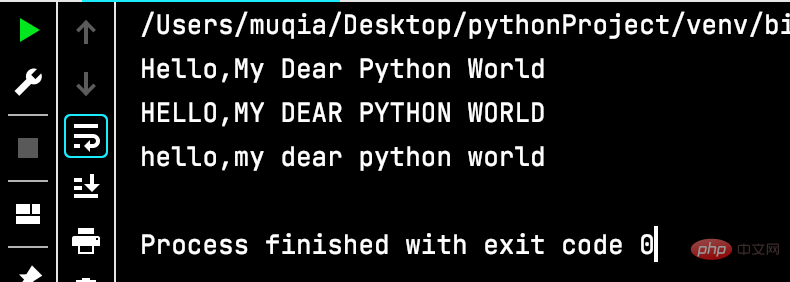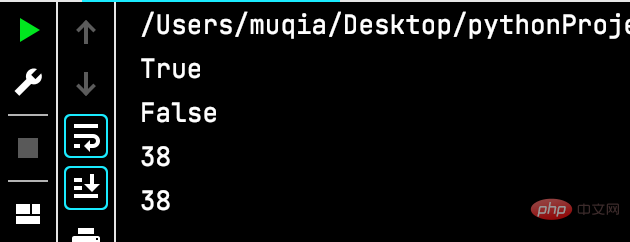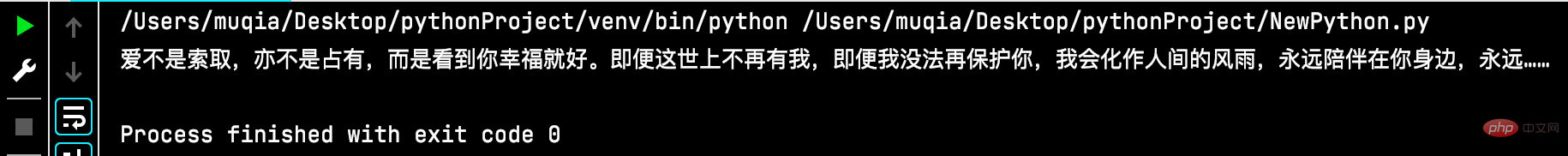# Python数据类型详解之字符串、数字## 变量

Python 中的变量不需要声明。每个变量在使用前都必须赋值，变量赋值以后该变量才会被创建。

```message = “hello,Python"
print(message)```Python允许你同时为多个变量赋值。如：

```a = b = c = 1
a, b, c = 1, 2, "runoob"```

## 变量的命名规则

1. 变量名只能包含字母、数字和下划线。可以字母和下划线开头，但不能数字开头。
2. 变量名不能含有空格，但可以使用下划线来分割其中的单词。
3. 不能讲Python关键字用作变量名。Python 的标准库提供了一个 keyword 模块，可以输出当前版本的所有关键字：
```import keyword
print(keyword.kwlist)```Python中一般使用小写字母的变量名。在变量名中使用大写字母虽然不会导致错误，但还是应避免使用大写字母。

## 标准数据类型

Python3 中有六个标准的数据类型：

• Number（数字）
• String（字符串）
• List（列表）
• Tuple（元组）
• Set（集合）
• Dictionary（字典）

Python3 的六个标准数据类型中：

• 不可变数据（3 个）：Number（数字）、String（字符串）、Tuple（元组）；
• 可变数据（3 个）：List（列表）、Dictionary（字典）、Set（集合）。

## 字符串

### 字符串（string）

```word = '字符串'
sentence = "这是一个句子。"
paragraph = """这是一个段落，

• python中单引号和双引号使用完全相同。
• 使用三引号(’’’ 或 “”")可以指定一个多行字符串。
• 转义符 \。
• 反斜杠可以用来转义，使用r可以让反斜杠不发生转义。。 如 r"this is a line with \n" 则\n会显示，并不是换行。
• 按字面意义级联字符串，如"this " "is " "string"会被自动转换为this is string。
• 字符串可以用 + 运算符连接在一起，用 * 运算符重复。
• Python 中的字符串有两种索引方式，从左往右以 0 开始，从右往左以 -1 开始。
• Python中的字符串不能改变。
• Python 没有单独的字符类型，一个字符就是长度为 1 的字符串。
• 字符串的截取的语法格式如下：变量[头下标:尾下标:步长]
```str='史迪崽儿的Python日记'

print(str)                 # 输出字符串
print(str[0:-1])           # 输出第一个到倒数第二个的所有字符
print(str)              # 输出字符串第一个字符
print(str[2:5])            # 输出从第三个开始到第五个的字符
print(str[2:])             # 输出从第三个开始后的所有字符
print(str[1:5:2])          # 输出从第二个开始到第五个且每隔一个的字符（步长为2）
print(str * 2)             # 输出字符串两次
print(str + '你好')         # 连接字符串

print('------------------------------')

print('hello\nrunoob')      # 使用反斜杠(\)+n转义特殊字符
print(r'hello\nrunoob')     # 在字符串前面添加一个 r，表示原始字符串，不会发生转义

print('\n')       # 输出空行
print(r'\n')      # 输出 \n
>>>```### 转义字符

• 在字符串中添加制表符，“\t”。
• 在字符串中添加换行符，“\n”。
• 反斜杠符号，“\”。
• 单引号，“’”。
• 双引号，“"”。
• 回车，“\r”。
```str1 = "睡觉诗"
str2 = "\'史迪崽儿\'\t2021-12-13"
str3 = "春困秋乏夏打盹\n冬眠不是一小会"
print(str1)
print(str2)
print(str3)```### 修改字符串大小写

• 将每个单词的首字母都改为大写，title()。
• 将字母全部转换为大写，upper()。
• 将字母全部转换为小写，lower()。
```str = "hello,my dear Python world"
print(str.title())
print(str.upper())
print(str.lower())```### 删除字符串空白

• 去除末尾空白，rstrip()。
• 去除开头空白，lstrip()。
• 去除开头和末尾空白，strip()。
```str = "   A   "
print(str)
print(str.rstrip())
print(str.lstrip())
print(str.strip())```### 判断字符串全是字母或数字

• 判断字符串全是字母，isalpha()。
• 判断字符串全是数字，isdigit()。
• 判断字符串既有字母又有数字，isalnum()。
```str1 = "abc"
str2 = "123"
str3 = "abc123"
print("三个字符串是否全为字母：")
print(str1.isalpha())
print(str2.isalpha())
print(str3.isalpha())
print("三个字符串是否全为数字：")
print(str1.isdigit())
print(str2.isdigit())
print(str3.isdigit())
print("三个字符串是否既有字母又有数字：")
print(str1.isalnum())
print(str2.isalnum())
print(str3.isalnum())```### 字符串查找

• 首词的查找，startswith()。
• 尾词的查找，endswith()。
• 任意位置词的查找，从左往右查find()或从右往左查rfind()。
```names = "张三"
print(names.startswith("张"))
print(names.endswith("四"))
articles = "爱不是索取，亦不是占有，而是看到你幸福就好。即便这世上不再有我，即便我没法再守护你，我会化作人间的风雨，永远陪伴在你身边，永远……"
print(articles.find("守护"))
print(articles.rfind("守护"))```### 字符串替换

replace()，替换。

```articles = "爱不是索取，亦不是占有，而是看到你幸福就好。即便这世上不再有我，即便我没法再守护你，我会化作人间的风雨，永远陪伴在你身边，永远……"
print(articles.replace("守护","保护"))```## 数字（Number）

Python3 支持 int、float、bool、complex（复数）。

```a = 111
print(isinstance(a, int))```

isinstance 和 type 的区别在于：
type()不会认为子类是一种父类类型。
isinstance()会认为子类是一种父类类型。

**注意：**Python3 中，bool 是 int 的子类，True 和 False 可以和数字相加， True == 1、False == 0 会返回 True，但可以通过 is 来判断类型。

+加法
-减法
*乘法
/除法
%取余
//整除取商
**幂

### 赋值运算符

=赋值
+=加赋值
-+减赋值
*=乘赋值
/=除赋值
%=取余赋值
//=整除赋值
**=幂赋值

**注意：**在其他语言中，如C++，java中，都有自增自减操作符“++”，“–”，但是在Python中表示正负。1/1